SPSS TUTORIALS BASICS ANOVA REGRESSION FACTOR CORRELATION

How to Run Levene’s Test in SPSS?

Levene’s test examines if 2+ populations all have
equal variances on some variable.

Levene's Test - What Is It?

If we want to compare 2(+) groups on a quantitative variable, we usually want to know if they have equal mean scores. For finding out if that's the case, we often use

Both tests require the homogeneity assumption: the population variances of the dependent variable must equal for all groups. You can ignore this assumption if you have roughly equal sample sizes for each group. However, if you have sharply different sample sizes, then you do need to make sure that homogeneity of variances is met by your data.

Now, we usually don't know the population variances. However, we do know the sample variances. And if these don't differ too much, then the population variances being equal seems credible.

But at what point do we no longer believe the population variances to be all equal? Levene’s test tells us precisely that.

Null Hypothesis

The null hypothesis for Levene's test is that the groups we're comparing all have equal population variances. If this is true, we'll probably find slightly different variances in our samples from these populations. However, very different sample variances suggests that the population variances weren't equal after all. In this case we'll reject the null hypothesis of equal population variances.

Levene's Test - Assumptions

Levene's test basically requires two assumptions:

• independent observations and
• the test variable is quantitative -that is, not nominal or ordinal.

Levene's Test - Example

A fitness company wants to know if 2 supplements for stimlating body fat loss actually work. They test 2 supplements (a cortisol blocker and a thyroid booster) on 20 people each and another 40 people receive a placebo.
All 80 participants have body fat measurements at the start of the experiment (week 11) and weeks 14, 17 and 20. This results in fatloss_unequal.sav, part of which is shown below.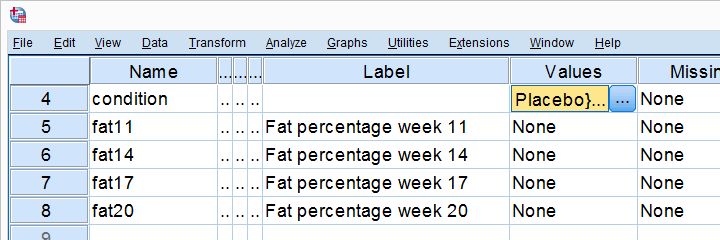One approach to these data is comparing body fat percentages over the 3 groups (placebo, thyroid, cortisol) for each week separately.Perhaps a better approach to these data is using a single repeated measures ANOVA. Weeks would be the within-subjects factor and supplement would be the between-subjects factor. For now, we'll leave it as an exercise to the reader to carry this out. We can use an ANOVA for each of the 4 body fat measurements. Since we've unequal sample sizes, we need to make sure that each supplement group has the same variance on each of the 4 measurements first.

Running Levene's test in SPSS

Several SPSS commands contain an option for running Levene's test. The easiest way to go -especially for multiple variables- is the One-Way ANOVA dialog.The main limitation of the One-Way ANOVA dialog is that it doesn't include any measures of effect size. For more on this, see How to Get (Partial) Eta Squared from SPSS?.
Right, so we navigate to AnalyzeCompare MeansOne-Way ANOVA and fill out the dialog that pops up.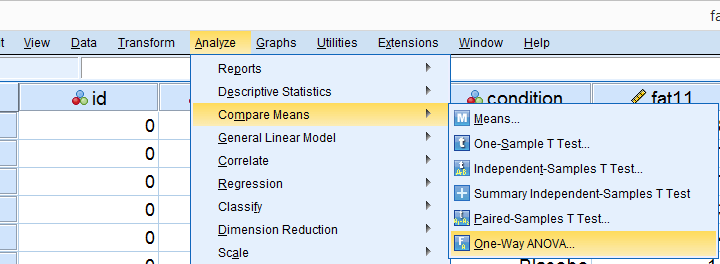As shown below, the Homogeneity of variance test under refers to Levene's test.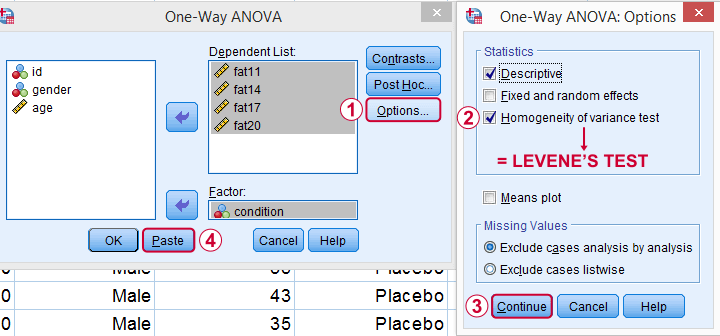Clicking results in the syntax below. Let's run it.

SPSS Levene's Test Syntax Example

*SPSS Levene's test syntax as pasted from Analyze - Compare Means - One-Way ANOVA.

ONEWAY fat11 fat14 fat17 fat20 BY condition
/STATISTICS DESCRIPTIVES HOMOGENEITY
/MISSING ANALYSIS.

Output for Levene's test

On running our syntax, we get several tables. The second -shown below- is the Test of Homogeneity of Variances. This holds the results of Levene's test.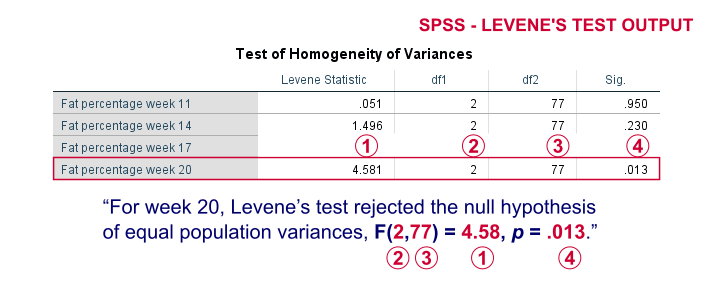As a rule of thumb, we conclude that population variances are not equal if “Sig.” or p < 0.05. For the first 2 variables, p > 0.05: for fat percentage in weeks 11 and 14 we don't reject the null hypothesis of equal population variances.
For the last 2 variables, p < 0.05: for fat percentages in weeks 17 and 20, we reject the null hypothesis of equal population variances. So these 2 variables violate the homogeity of variance assumption needed for an ANOVA.

Descriptive Statistics Output

Remember that we don't need equal population variances if we have roughly equal sample sizes. A sound way for evaluating if this holds is inspecting the Descriptives table in our output.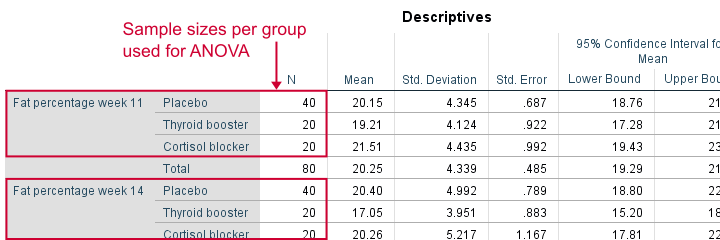As we see, our ANOVA is based on sample sizes of 40, 20 and 20 for all 4 dependent variables. Because they're not (roughly) equal, we do need the homogeneity of variance assumption but it's not met by 2 variables.
In this case, we'll report some alternative results (Welch and Games-Howell) but these are beyond the scope of this tutorial.

Reporting Levene's test

Perhaps surprisingly, Levene's test is technically an ANOVA as we'll explain here. We therefore report it like a basic ANOVA too. So we'll write something like “Levene’s test showed that the variances for body fat percentage in week 20 were not equal, F(2,77) = 4.58, p = 0.013.”

Levene's Test - How Does It Work?

Levene's test works very simply: a larger variance means that -on average- the data values are “further away” from their mean. The figure below illustrates this: watch the histograms become “wider” as the variances increase.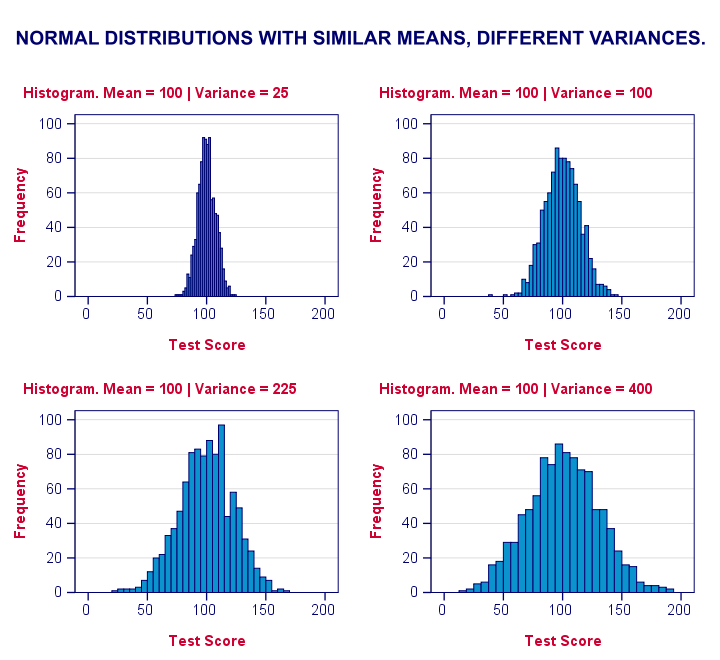We therefore compute the absolute differences between all scores and their (group) mean. The means of the absolute differences should be roughly equal over groups. So technically, Levene's test is an ANOVA on the absolute differences. In other words: we run an ANOVA (on absolute differences) to find out if we can run an ANOVA (on our actual data).
If that just sounds too weird, then try running the syntax below. It does exactly what I just explained.

“Manual” Levene's Test Syntax

*Add group means on fat20 to dataset.

/break condition
/mfat20 = mean(fat20).

*Compute absolute differences between fat20 and group means.

compute adfat20 = abs(fat20 - mfat20).

*Run minimal ANOVA on absolute differences. F-test identical to previous Levene's test.

Result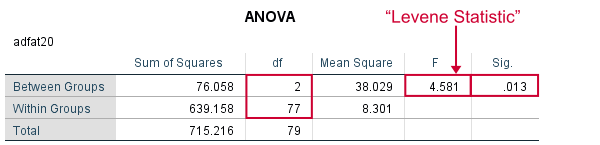As we see, these ANOVA results are identical to Levene's test in the previous output. I hope this clarifies why we report it as an ANOVA as well.

Tell us what you think!

*Required field. Your comment will show up after approval from a moderator.

•By Ruben Geert van den Berg on April 17th, 2020

Hi Robin!

Good question. It's the "normal" Levene's test based on the mean that you're looking for.

Note that ANOVA via the Oneway dialog includes "Brown-Forsythe" and "Welch". Both are alternatives for the usual F-test that do not require homogeneity.

Hope that helps!

SPSS tutorials

•By Robin on April 17th, 2020

•By Cynthia M. Terrell on May 30th, 2020

Very good demonstration and information does help.

•By Obinna on December 16th, 2020

Well explained in a simple language, especially for beginners. Thanks so much.

•By Akram Sadeghi on May 7th, 2021

It was very helpful, thank you so much